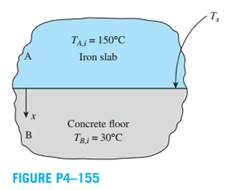Chat Now

# A large iron slab

### A large iron slab

A large iron slab (ρ = 7870 kg/m3 , cp = 447 J/kg·K, and k = 80.2 W/m·K) was initially heated to a uniform temperature of 150°C and then placed on concrete floor (ρ = 1600 kg/m3 , cp = 840 J/kg·K, and k = 0.79 W/m·K). The concrete floor was initially at a uniform temperature of 30°C. Determine

(a) The surface temperature between the iron slab and concrete floor and

(b) The temperature of the concrete floor at the depth of 25 mm, if the surface temperature remains constant after 15 minutes.rahul 20-Jul-2020Get solution